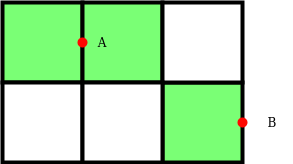# Minimum number of points required to cover all blocks of a 2-D grid

• Difficulty Level : Easy
• Last Updated : 11 May, 2022

Given two integers N and M. The task is to find the minimum number of points required to cover an N * M grid.

A point can cover two blocks in a 2-D grid when placed in any common line or sideline.

Examples:

Input: N = 5, M = 7
Output: 18
Input: N = 3, M = 8
Output: 12

Approach: This problem can be solved using Greedy Approach. The main idea is to observe that a single point placed on the common line or sideline covers two blocks. So the total number of points needed to cover all the blocks(say B blocks) is B/2 when B is even else B/2 + 1 when B is odd.
For a grid having N*M blocks, The total number of blocks will be (N*M)/2 when either one of them is even. Otherwise, it will require ((N*M)/2) + 1 points to cover all the blocks and one extra for last untouched block.
Below is the image to show how points can be used to cover block in a 2D-grid:Point ‘A’ covers two blocks and ‘B’ covers one block.
Below is the implementation of the above approach:

## C++

 `// C++ program for the above approach``#include ``using` `namespace` `std;` `// Function to find the minimum number``// of Points required to cover a grid``int` `minPoints(``int` `n, ``int` `m)``{``    ``int` `ans = 0;` `    ``// If number of block is even``    ``if` `((n % 2 != 0)``        ``&& (m % 2 != 0)) {``        ``ans = ((n * m) / 2) + 1;``    ``}``    ``else` `{``        ``ans = (n * m) / 2;``    ``}` `    ``// Return the minimum points``    ``return` `ans;``}` `// Driver Code``int` `main()``{``    ``// Given size of grid``    ``int` `N = 5, M = 7;` `    ``// Function Call``    ``cout << minPoints(N, M);``    ``return` `0;``}`

## Java

 `// Java program for the above approach``class` `GFG{``    ` `// Function to find the minimum number``// of Points required to cover a grid``static` `int` `minPoints(``int` `n, ``int` `m)``{``    ``int` `ans = ``0``;` `    ``// If number of block is even``    ``if` `((n % ``2` `!= ``0``) && (m % ``2` `!= ``0``))``    ``{``        ``ans = ((n * m) / ``2``) + ``1``;``    ``}``    ``else``    ``{``        ``ans = (n * m) / ``2``;``    ``}` `    ``// Return the minimum points``    ``return` `ans;``}` `// Driver Code``public` `static` `void` `main (String[] args)``{``    ``// Given size of grid``    ``int` `N = ``5``, M = ``7``;` `    ``// Function Call``    ``System.out.print(minPoints(N, M));``}``}` `// This code is contributed by Ritik Bansal`

## Python3

 `# Python3 program for the above approach` `# Function to find the minimum number``# of Points required to cover a grid``def` `minPoints(n, m):` `    ``ans ``=` `0` `    ``# If number of block is even``    ``if` `((n ``%` `2` `!``=` `0``) ``and` `(m ``%` `2` `!``=` `0``)):``        ``ans ``=` `((n ``*` `m) ``/``/` `2``) ``+` `1` `    ``else``:``        ``ans ``=` `(n ``*` `m) ``/``/` `2` `    ``# Return the minimum points``    ``return` `ans` `# Driver code``if` `__name__ ``=``=` `'__main__'``:` `    ``# Given size of grid``    ``N ``=` `5``    ``M ``=` `7` `    ``# Function call``    ``print``(minPoints(N, M))` `# This code is contributed by himanshu77`

## C#

 `// C# program for the above approach``using` `System;``class` `GFG{``    ` `// Function to find the minimum number``// of Points required to cover a grid``static` `int` `minPoints(``int` `n, ``int` `m)``{``    ``int` `ans = 0;` `    ``// If number of block is even``    ``if` `((n % 2 != 0) && (m % 2 != 0))``    ``{``        ``ans = ((n * m) / 2) + 1;``    ``}``    ``else``    ``{``        ``ans = (n * m) / 2;``    ``}` `    ``// Return the minimum points``    ``return` `ans;``}` `// Driver Code``public` `static` `void` `Main(String[] args)``{``    ``// Given size of grid``    ``int` `N = 5, M = 7;` `    ``// Function Call``    ``Console.Write(minPoints(N, M));``}``}` `// This code is contributed by sapnasingh4991`

## Javascript

 ``

Output:

`18`

Time Complexity: O(1)
Auxiliary Space: O(1)

My Personal Notes arrow_drop_up